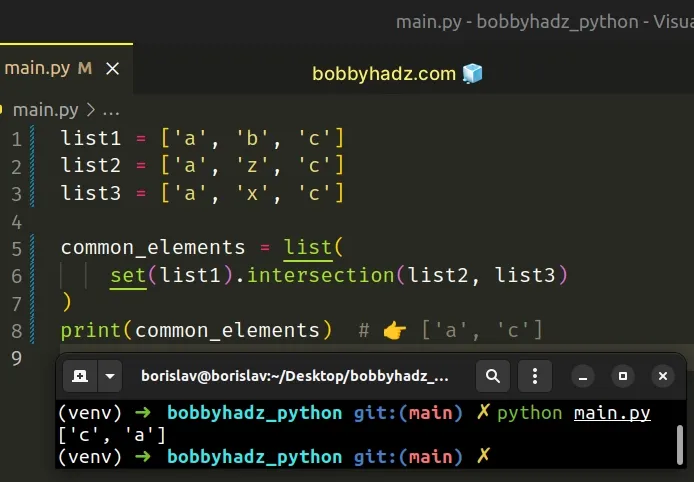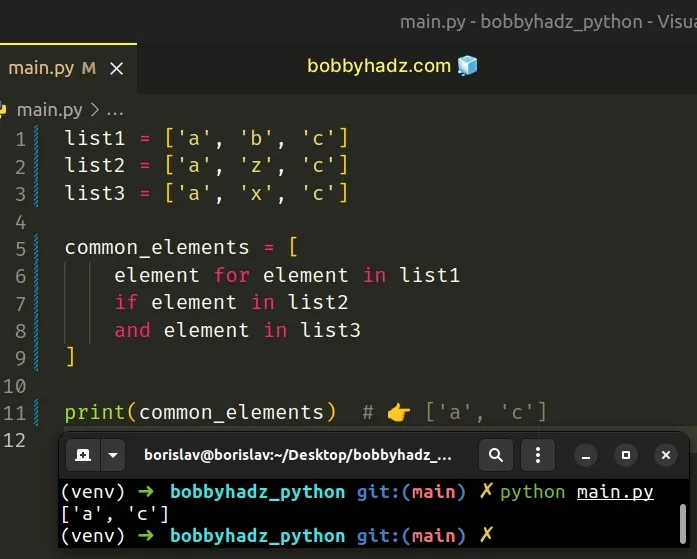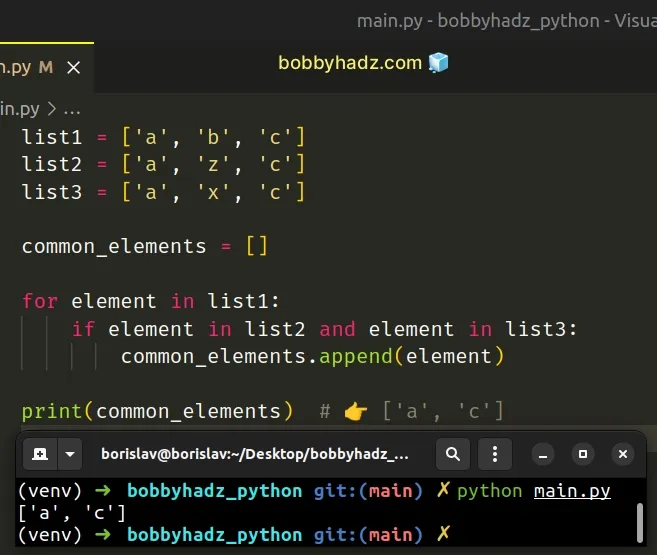# Find common values in multiple Lists in PythonLast updated: Feb 22, 2023
5 min## #Find common values in multiple lists in Python

To find the common values in multiple lists:

1. Convert the first list to a `set` object.
2. Use the `intersection()` method on the `set`.
3. The `intersection` method will return the common elements in the lists.
main.py
```Copied!```list1 = ['a', 'b', 'c']
list2 = ['a', 'z', 'c']
list3 = ['a', 'x', 'c']

common_elements = list(
set(list1).intersection(list2, list3)
)
print(common_elements)  # 👉️ ['a', 'c']
``````If you need to find the common elements in a list of lists, click on the following subheading:

The example finds the common elements in three lists, but you can use this approach with as many lists as necessary.

We used the `set()` class to convert the first list to a `set`.

The set() class takes an iterable optional argument and returns a new `set` object with elements taken from the iterable.

main.py
```Copied!```list1 = ['a', 'b', 'c']

print(set(list1))  # 👉️ {'a', 'b', 'c'}
``````
Set objects store an unordered collection of unique elements and implement an `intersection()` method.

The intersection method returns a new `set` with elements common to the provided objects.

main.py
```Copied!```list1 = ['a', 'b', 'c']
list2 = ['a', 'z', 'c']
list3 = ['a', 'x', 'c']

common_elements = list(
set(list1).intersection(list2, list3)
)
print(common_elements)  # 👉️ ['a', 'c']

print(len(common_elements))  # 👉️ 2
``````
You can use the `len()` function if you need to get the number of common elements between the lists.

Alternatively, you can use a list comprehension.

## #Find common values in multiple lists using a list comprehension

This is a three-step process:

1. Use a list comprehension to iterate over the first list.
2. Check if each element is present in the other lists and return the result.
3. The new list will only contain the common elements between the lists.
main.py
```Copied!```list1 = ['a', 'b', 'c']
list2 = ['a', 'z', 'c']
list3 = ['a', 'x', 'c']

common_elements = [
element for element in list1
if element in list2
and element in list3
]

print(common_elements)  # 👉️ ['a', 'c']
``````We used a list comprehension to iterate over the first list.

List comprehensions are used to perform some operation for every element or select a subset of elements that meet a condition.

On each iteration, we check if the current item is present in the two other lists and return the result.

The new list only contains the elements that are present in all three lists.

The in operator tests for membership. For example, `x in l` evaluates to `True` if `x` is a member of `l`, otherwise, it evaluates to `False`.

Alternatively, you can use a simple for loop.

## #Find common values in multiple lists using a for loop

This is a three-step process:

1. Use a `for` loop to iterate over the first list.
2. Check if each item is contained in the other lists.
3. Append the matching items to a new list.
main.py
```Copied!```list1 = ['a', 'b', 'c']
list2 = ['a', 'z', 'c']
list3 = ['a', 'x', 'c']

common_elements = []

for element in list1:
if element in list2 and element in list3:
common_elements.append(element)

print(common_elements)  # 👉️ ['a', 'c']
``````We used a `for` loop to iterate over the first list.

On each iteration, we use the `in` operator to check if the current item is contained in the other two lists.

If the condition is met, we append the value to a new list.

The list.append() method adds an item to the end of the list.

main.py
```Copied!```my_list = ['bobby', 'hadz']

my_list.append('com')

print(my_list)  # 👉️ ['bobby', 'hadz', 'com']
``````

The method returns None as it mutates the original list.

## #Find the common elements in a List of Lists in Python

To find the common elements in a list of lists:

1. Convert the first element in the list to a `set`.
2. Call the `intersection()` method on the `set`.
3. The method will return the common elements in the list of lists.
main.py
```Copied!```list_of_lists = [
[4, 7, 9],
[4, 9, 9],
[4, 1, 9]
]

common_elements = set(
list_of_lists
).intersection(*list_of_lists)

print(common_elements)  # 👉️ {9, 4}

print(list(common_elements))  # 👉️ [9, 4]
``````

We used the `set()` class to convert the first nested list to a `set` object.

main.py
```Copied!```list_of_lists = [
[4, 7, 9],
[4, 9, 9],
[4, 1, 9]
]

print(set(list_of_lists)) # 👉️ {9, 4, 7}
``````
Set objects store an unordered collection of unique elements and implement an`intersection()` method.

The intersection method returns a new `set` with elements common to both `set` objects.

main.py
```Copied!```list_of_lists = [
[4, 7, 9],
[4, 9, 9],
[4, 1, 9]
]

common_elements = set(
list_of_lists
).intersection(*list_of_lists)

print(common_elements)  # 👉️ {9, 4}

print(list(common_elements))  # 👉️ [9, 4]
``````

We called the `intersection()` method on the `set` and used the iterable unpacking operator to unpack the list of lists in the call to the method.

The * iterable unpacking operator enables us to unpack an iterable in function calls, in comprehensions and in generator expressions.

You can imagine that the nested lists got passed as multiple, comma-separated arguments to the `intersection` method.

The `intersection` method then returned a `set` object containing the common elements in the lists.

Use the `list()` class if you need to convert the `set` to a list.

The list class takes an iterable and returns a list object.

Alternatively, you can use the `intersection_update()` method.

## #Find the common elements in a List of Lists using intersection_update()

This is a three-step process:

1. Convert the first element in the list to a `set`.
2. Use a `for` loop to iterate over the rest of the list.
3. Use the `intersection_update` method to find the common elements.
main.py
```Copied!```list_of_lists = [
[4, 7, 9],
[4, 9, 9],
[4, 1, 9]
]

common_elements = set(list_of_lists)

for l in list_of_lists[1:]:
common_elements.intersection_update(l)

print(common_elements)  # {9, 4}
``````

We converted the first nested list to a `set` and stored the result in a variable.

We then used a `for` loop to iterate over the rest of the list.

The slice `list_of_lists[1:]` starts at index `1` and goes to the end of the list.

On each iteration, we use the `intersection_update` method to update the set with the intersection of itself and the list.

The intersection_update method updates the `set` in place.

After the last iteration, the variable stores the common elements in the list of lists.